# Checking If Matching Numbers Exist in Different Ranges

In this article, you’ll learn how to check if matching numbers exist in different ranges in Microsoft Excel.

When we want to pick the values from first data to second data, we use Vlookup function. But, when Vlookup function does not get the common values in second data, Vlookup gives #N/A error. To avoid the #N/A error, we can use IFNA function.

• Vlookup function helps to pick the value from 1st data to 2nd data.
• IFNA function helps to define the value at the place of #N/A error.

Let’s take an example and understand how we can use these formulas to get the result.

We have 2 data sets. 1st is main data and 2nd is updated data. Now, we want to update the main data from the updated data.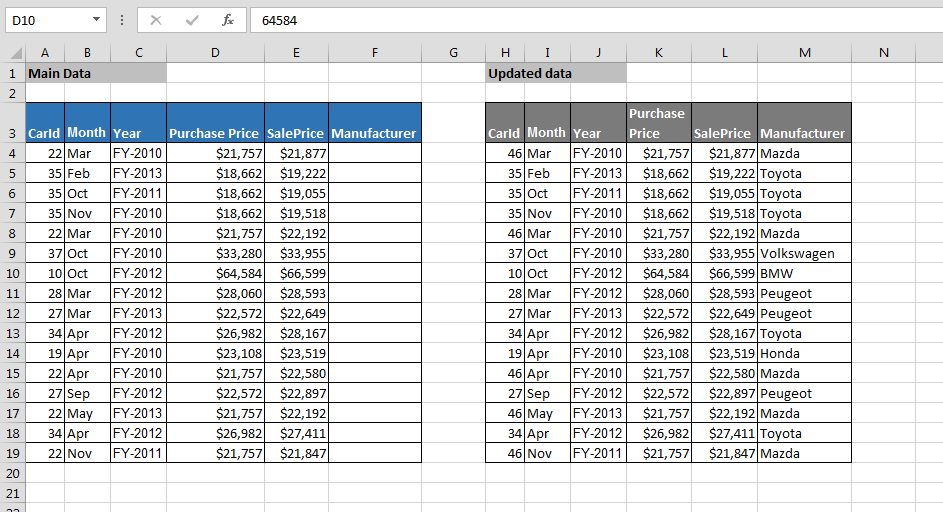Main Data:-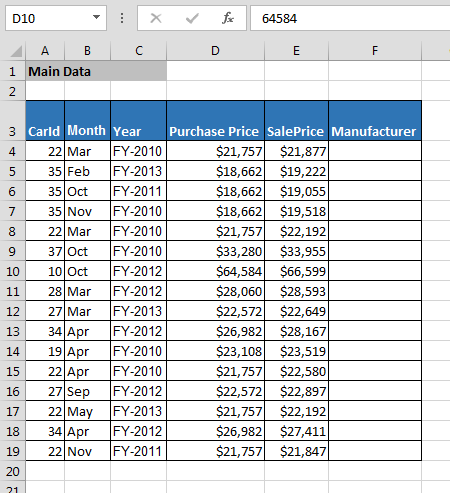Updated Data:-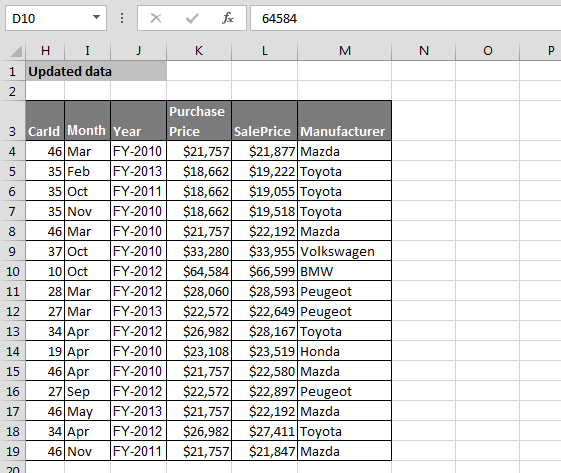In the main data, we want to update the manufacturer details from the updated sheet.

• Enter the formula in cell F4 of Main data
• Press Enter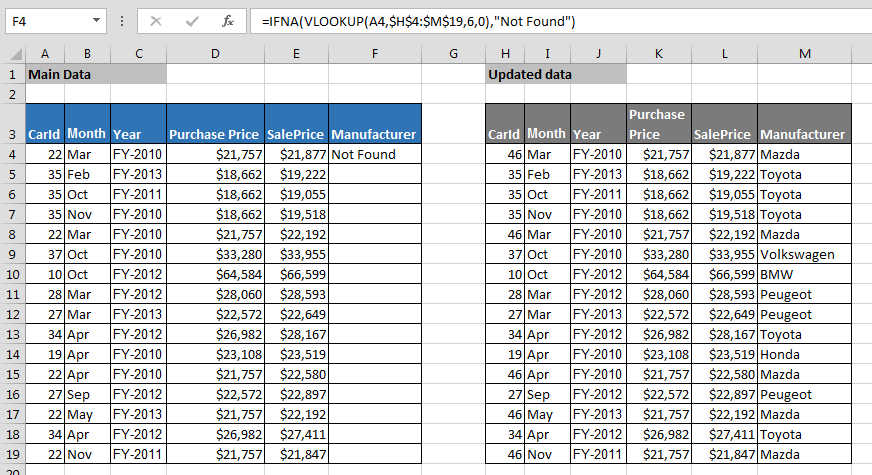• Copy the same formula and paste in the range.
• Manufacturer detail will get updated, and also, where the data is not available in the sheet, formula will return “Not Found” as output.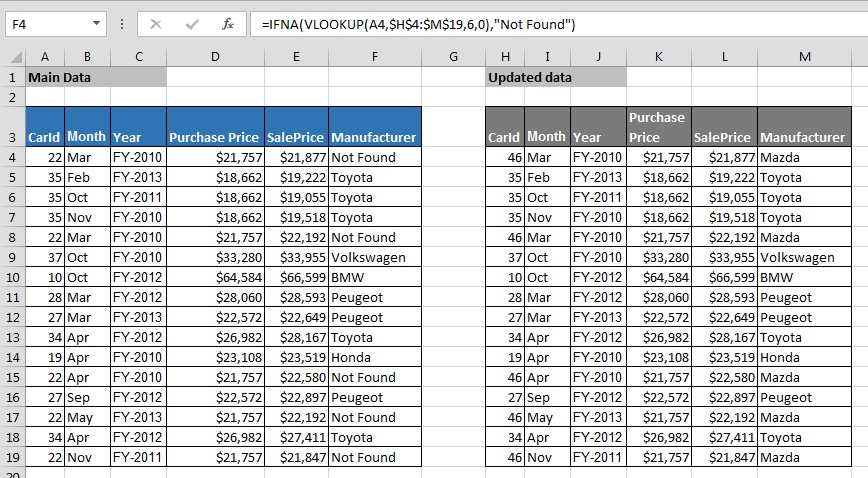In this way, we can ignore #N/A error by using IFNA function in Microsoft Excel. Also, this function is compatible with 2007 and later versions of Microsoft Excel.

If you are using 2003 Microsoft Excel, then you can use below given function:-